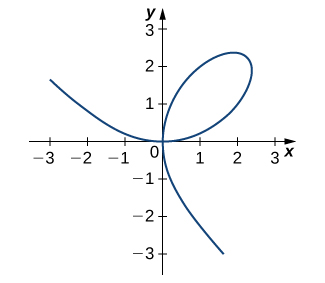$$\newcommand{\id}{\mathrm{id}}$$ $$\newcommand{\Span}{\mathrm{span}}$$ $$\newcommand{\kernel}{\mathrm{null}\,}$$ $$\newcommand{\range}{\mathrm{range}\,}$$ $$\newcommand{\RealPart}{\mathrm{Re}}$$ $$\newcommand{\ImaginaryPart}{\mathrm{Im}}$$ $$\newcommand{\Argument}{\mathrm{Arg}}$$ $$\newcommand{\norm}{\| #1 \|}$$ $$\newcommand{\inner}{\langle #1, #2 \rangle}$$ $$\newcommand{\Span}{\mathrm{span}}$$

# 2.6E: Exercises

$$\newcommand{\vecs}{\overset { \rightharpoonup} {\mathbf{#1}} }$$ $$\newcommand{\vecd}{\overset{-\!-\!\rightharpoonup}{\vphantom{a}\smash {#1}}}$$$$\newcommand{\id}{\mathrm{id}}$$ $$\newcommand{\Span}{\mathrm{span}}$$ $$\newcommand{\kernel}{\mathrm{null}\,}$$ $$\newcommand{\range}{\mathrm{range}\,}$$ $$\newcommand{\RealPart}{\mathrm{Re}}$$ $$\newcommand{\ImaginaryPart}{\mathrm{Im}}$$ $$\newcommand{\Argument}{\mathrm{Arg}}$$ $$\newcommand{\norm}{\| #1 \|}$$ $$\newcommand{\inner}{\langle #1, #2 \rangle}$$ $$\newcommand{\Span}{\mathrm{span}}$$ $$\newcommand{\id}{\mathrm{id}}$$ $$\newcommand{\Span}{\mathrm{span}}$$ $$\newcommand{\kernel}{\mathrm{null}\,}$$ $$\newcommand{\range}{\mathrm{range}\,}$$ $$\newcommand{\RealPart}{\mathrm{Re}}$$ $$\newcommand{\ImaginaryPart}{\mathrm{Im}}$$ $$\newcommand{\Argument}{\mathrm{Arg}}$$ $$\newcommand{\norm}{\| #1 \|}$$ $$\newcommand{\inner}{\langle #1, #2 \rangle}$$ $$\newcommand{\Span}{\mathrm{span}}$$

## Exercise $$\PageIndex{1}$$

For the following exercises, use implicit differentiation to find $$\frac{dy}{dx}$$.

1) $$x^2−y^2=4$$

2) $$6x^2+3y^2=12$$

3) $$x^2y=y−7$$

4) $$3x^3+9xy^2=5x^3$$

5) $$xy−cos(xy)=1$$

6) $$y\sqrt{x+4}=xy+8$$

7) $$−xy−2=\frac{x}{7}$$

8) $$ysin(xy)=y^2+2$$

9) $$(xy)^2+3x=y^2$$

10) $$x^3y+xy^3=−8$$

1. $$\frac{dy}{dx}=\frac{x}{y}$$

2. $$\frac{dy}{dx}=\frac{−2x}{y}$$

3. $$\frac{dy}{dx}=\frac{-2xy}{x^2-1}$$

4. $$\frac{dy}{dx}=\frac{x}{3y}−\frac{y}{2x}$$

5. $$\frac{dy}{dx}=\frac{-y}{x}$$

6. $$\frac{dy}{dx}=\frac{y−\frac{y}{2\sqrt{x+4}}}{\sqrt{x+4}−x}$$

7. $$\frac{dy}{dx}=-\frac{1+7y}{7x}$$

8. $$\frac{dy}{dx}=\frac{y^2cos(xy)}{2y−sin(xy)−xycosxy}$$

9. $$\frac{dy}{dx}=\frac{-2xy^2-3}{2y(x^2-1)}$$

10. $$\frac{dy}{dx}=\frac{−3x^2y−y^3}{x^3+3xy^2}$$

Solution

1. $$x^2−y^2=4$$

$$2x-2y(\frac{dy}{dx})=0$$

$$2y(\frac{dy}{dx})=2x$$

$$\frac{dy}{dx}=\frac{2x}{2y}$$

$$\frac{dy}{dx}=\frac{x}{y}$$

2. $$6x^2+3y^2=12$$

$$12x+6y(\frac{dy}{dx})=0$$

$$6y(\frac{dy}{dx})=-12x$$

$$\frac{dy}{dx}=\frac{-12x}{6y}$$

$$\frac{dy}{dx}=\frac{-2x}{y}$$

3. $$x^2y=y−7$$

$$2xy+x^2(\frac{dy}{dx})=\frac{dy}{dx}-0$$

$$x^2(\frac{dy}{dx})-\frac{dy}{dx}=-2xy$$

$$\frac{dy}{dx}(x^2-1)=-2xy$$

$$\frac{dy}{dx}=\frac{-2xy}{x^2-1}$$

4. $$3x^3+9xy^2=5x^3$$

$$9x^2+9y^2+18xy(\frac{dy}{dx})=15x^2$$

$$18xy(\frac{dy}{dx})=3x^2-9y^2$$

$$\frac{dy}{dx}=\frac{3x^2-9y^2}{18xy}$$

$$\frac{dy}{dx}=\frac{x}{3y}-\frac{y}{2x}$$

5. $$xy−cos(xy)=1$$

$$x(\frac{dy}{dx})+y+sin(xy)(x(\frac{dy}{dx})+y)=0$$

$$x(\frac{dy}{dx})+x(\frac{dy}{dx})sin(xy)+ysin(xy)=-y$$

$$x\frac{dy}{dx}(1+sin(xy))=-y-ysin(xy)$$

$$x\frac{dy}{dx}(1+sin(xy))=-y(1+sin(xy))$$

$$x\frac{dy}{dx}=-y$$

$$\frac{dy}{dx}=\frac{-y}{x}$$

6. $$y\sqrt{x+4}=xy+8$$

$$y(x+4)^(1/2)=xy+8$$

$$\frac{1}{2}y(x+4)^(-1/2)+\frac{dy}{dx}(x+4)^(1/2)=x\frac{dy}{dx}+y=0$$

$$\frac{dy}{dx}\sqrt{x+4}-x\frac{dy}{dx}=y-\frac{y}{2\sqrt{x+4}}$$

$$\frac{dy}{dx}(\sqrt{x+4}-x)=y-\frac{y}{2\sqrt{x+4}}$$

$$\frac{dy}{dx}=\frac{y-\frac{y}{2\sqrt{x+4}}}{\sqrt{x+4}-x}$$

7. $$−xy−2=\frac{x}{7}$$

$$-x\frac{dy}{dx}-y-0=\frac{1}{7}$$

$$-x\frac{dy}{dx}=\frac{1}{7}+y$$

$$\frac{dy}{dx}=\frac{\frac{1}{7}+y}{-x}$$

$$\frac{dy}{dx}=-\frac{1+7y}{7x}$$

8. $$ysin(xy)=y^2+2$$

$$ycos(xy)(x\frac{dy}{dx}+y)+\frac{dy}{dx}sin(xy)=2y\frac{dy}{dx}$$

$$\frac{dy}{dx}xycos(xy)+y^2cos(xy)+\frac{dy}{dx}sin(xy)-2y\frac{dy}{dx}=0$$

$$\frac{dy}{dx}xycos(xy)+\frac{dy}{dx}sin(xy)-2y\frac{dy}{dx}=-y^2cos(xy)$$

$$\frac{dy}{dx}(xycos(xy)+sin(xy)-2y)=-y^2cos(xy)$$

$$\frac{dy}{dx}=\frac{-y^2cos(xy)}{-2y+sin(xy)+xycos(xy)}$$

$$\frac{dy}{dx}=\frac{y^2cos(xy)}{2y−sin(xy)−xycosxy}$$

9. $$(xy)^2+3x=y^2$$

$$2xy(x\frac{dy}{dx}+y)+3=2y\frac{dy}{dx}$$

$$2x^2y\frac{dy}{dx}+2xy^2-2y\frac{dy}{dx}+3=0$$

$$2x^2y\frac{dy}{dx}+2xy^2-2y\frac{dy}{dx}+3=0$$

$$\frac{dy}{dx}(2x^2y-2y)=-2xy^2-3$$

$$\frac{dy}{dx}=\frac{-2xy^2-3}{2y(x^2-1)}$$

10. $$x^3y+xy^3=−8$$

$$3x^2y+x^3\frac{dy}{dx}+y^3+3xy^2\frac{dy}{dx}=0$$

$$x^3\frac{dy}{dx}+3xy^2\frac{dy}{dx}=-3x^2y-y^3$$

$$\frac{dy}{dx}(x^3+3xy^2)=-3x^2y-y^3$$

$$\frac{dy}{dx}=\frac{-3x^2y-y^3}{x^3+3xy^2}$$

## Exercise $$\PageIndex{2}$$

For the following exercises, find the equation of the tangent line to the graph of the given equation at the indicated point. Use a calculator or computer software to graph the function and the tangent line.

1) $$x^4y−xy^3=−2,(−1,−1)$$

2) $$x^2y^2+5xy=14,(2,1)$$

3) $$xy^2+sin(πy)−2x^2=10,(2,−3)$$

4) $$\frac{x}{y}+5x−7=−\frac{3}{4}y,(1,2)$$

5) $$xy+sin(x)=1,(\frac{π}{2},0)$$

1.$$y=-\frac{5}{4}x-\frac{9}{4}$$

2. $$y=−\frac{1}{2}x+2$$

3. $$y=\frac{1}{π+12}x−\frac{3π+38}{π+12}$$

4.$$y=-\frac{11}{2}x+\frac{15}{2}$$

5. $$y=0$$\

Solution

1. $$x^4y−xy^3=−2,(−1,−1)$$

Take the derivative:

$$4x^3y+x^4\frac{dy}{dx}-y^3-3xy^2\frac{dy}{dx}=0$$

Input x and y values to solve for $$\frac{dy}{dx}$$ (this will be the slope of the tangent line):

$$4(-1)^3(-1)+(-1)^4\frac{dy}{dx}-(-1)^3-3(-1)(-1)^2\frac{dy}{dx}=0$$

$$4+\frac{dy}{dx}+1+3\frac{dy}{dx}=0$$

$$\frac{dy}{dx} (1+3)=-4-1$$

$$\frac{dy}{dx}=\frac{-5}{4}$$

Now use this slope and the x and y values provided to solve the slope point intercept equation:

$$y=\frac{-5}{4}x+b$$

$$-1=\frac{-5}{4}(-1)+b$$

$$-1=\frac{5}{4}+b$$

$$-1-\frac{5}{4}=b$$

$$b=\frac{-9}{4}$$

Therefore the equation of the tangent line is:

$$y=-\frac{5}{4}x-\frac{9}{4}$$

2. $$x^2y^2+5xy=14,(2,1)$$

Take the derivative:

$$2xy^2+2yx^2\frac{dy}{dx}+5y+5x\frac{dy}{dx}=0$$

Input x and y values to solve for $$\frac{dy}{dx}$$ (this will be the slope of the tangent line):

$$2(2)(1)^2+2(1)(2)^2\frac{dy}{dx}+5(1)+5(2)\frac{dy}{dx}=0$$

$$4+8\frac{dy}{dx}+5+10\frac{dy}{dx}=0$$

$$18\frac{dy}{dx}=-9$$

$$\frac{dy}{dx}=\frac{-1}{2}$$

Now use this slope and the x and y values provided to solve the slope point intercept equation:

$$y=\frac{-1}{2}x+b$$

$$1=\frac{-1}{2}(2)+b$$

$$1=-1+b$$

2=b

Therefore the equation of the tangent line is:

$$y=\frac{-1}{2}+2$$

3. $$xy^2+sin(πy)−2x^2=10,(2,−3)$$

Take the derivative:

$$y^2+2xy\frac{dy}{dx}+\pi cos(\pi y) \frac{dy}{dx} -4x=0$$

Input x and y values to solve for $$\frac{dy}{dx}$$ (this will be the slope of the tangent line):

$$(-3)^2+2(2)(-3)\frac{dy}{dx}+\pi cos(-3\pi)\frac{dy}{dx}-4(2)=0$$

$$9-12\frac{dy}{dx}0-\pi \frac{dy}{dx}-8=0$$

$$-12-\pi(\frac{dy}{dx})=-1$$

$$\frac{dy}{dx}=\frac{1}{12+\pi}$$

Now use this slope and the x and y values provided to solve the slope point intercept equation:

$$y=\frac{1}{12+ \pi}x+b$$

$$-3=(\frac{1}{12+ \pi})2+b$$

$$\frac{-3(12+\pi)-2}{12+ \pi}=b$$

$$\frac{-38-3\pi}{12+\pi}=b$$

Therefore the equation of the tangent line is:

$$y=\frac{1}{π+12}x−\frac{3π+38}{π+12}$$

## Exercise $$\PageIndex{3}$$

The graph of a folium of Descartes with equation $$2x^3+2y^3−9xy=0$$ is given in the following graph.a. Find the equation of the tangent line at the point $$(2,1)$$. Graph the tangent line along with the folium.

b. Find the equation of the normal line to the tangent line in a. at the point $$(2,1)$$.

Under Construction

## Exercise $$\PageIndex{4}$$

For the equation $$x^2+2xy−3y^2=0,$$

a. Find the equation of the normal to the tangent line at the point $$(1,1)$$.

b. At what other point does the normal line in a. intersect the graph of the equation?

a. $$y=−x+2$$

b. $$(3,−1)$$

## Exercise $$\PageIndex{5}$$

Find all points on the graph of $$y^3−27y=x^2−90$$ at which the tangent line is vertical.

For the equation $$x^2+xy+y^2=7$$,

a. Find the $$x$$-intercept(s).

b.Find the slope of the tangent line(s) at the x-intercept(s).

c. What does the value(s) in b. indicate about the tangent line(s)?

a. $$(±7√,0)$$

b. $$−2$$

c. They are parallel since the slope is the same at both intercepts.

## Exercise $$\PageIndex{6}$$

Find the equation of the tangent line to the graph of the equation $$sin^{−1x}+sin^{−1}y=\frac{π}{6}$$ at the point $$(0,\frac{1}{2})$$.

Under Construction

## Exercise $$\PageIndex{7}$$

For the automatic number to work, you need to add the "AutoNum" template (preferably at the end) to the page.

$$y=−x+1$$

## Exercise $$\PageIndex{8}$$

Find $$y′$$ and $$y''$$ for $$x^2+6xy−2y^2=3$$.

Under Construction

## Exercise $$\PageIndex{9}$$

The number of cell phones produced when $$x$$ dollars is spent on labor and $$y$$ dollars is spent on capital invested by a manufacturer can be modeled by the equation $$60x^{3/4}y^{1/4}=3240$$.

a. Find $$\frac{dy}{dx}$$ and evaluate at the point $$(81,16)$$.

b. Interpret the result of a.

a. $$−0.5926$$

b. When $81 is spent on labor and$16 is spent on capital, the amount spent on capital is decreasing by $0.5926 per$1 spent on labor.

## Exercise $$\PageIndex{10}$$

The number of cars produced when x dollars is spent on labor and y dollars is spent on capital invested by a manufacturer can be modeled by the equation $$30x^{1/3}y^{2/3}=360$$.

(Both $$x$$and $$y$$ are measured in thousands of dollars.)

a. Find $$\frac{dy}{dx}$$ and evaluate at the point $$(27,8)$$.

b. Interpret the result of a.

Under Construction

## Exercise $$\PageIndex{11}$$

The volume of a right circular cone of radius $$x$$ and height $$y$$ is given by $$V=\frac{1}{3}πx^2y$$. Suppose that the volume of the cone is $$85πcm^3$$. Find $$\frac{dy}{dx}$$ when $$x=4$$ and $$y=16$$.

$$−8$$

## Exercise $$\PageIndex{12}$$

For the following exercises, consider a closed rectangular box with a square base with side $$x$$ and height $$y$$.

1) Find an equation for the surface area of the rectangular box, $$S(x,y)$$.

2) If the surface area of the rectangular box is 78 square feet, find $$\frac{dy}{dx}$$ when $$x=3$$ feet and $$y=5$$ feet.

2. $$−2.67$$

## Exercise $$\PageIndex{13}$$

For the following exercises, use implicit differentiation to determine $$y′$$. Does the answer agree with the formulas we have previously determined?

1) $$x=siny$$

2) $$x=cosy$$

3) $$x=tany$$

2. $$y′=−\frac{1}{\sqrt{1−x^2}}$$